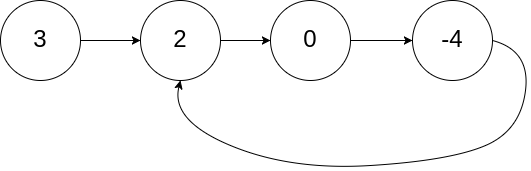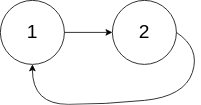# LeetCode: 141. Linked List Cycle

## 题目¶

Given head, the head of a linked list, determine if the linked list has a cycle in it.

There is a cycle in a linked list if there is some node in the list that can be reached again by continuously following the next pointer. Internally, pos is used to denote the index of the node that tail's next pointer is connected to. Note that pos is not passed as a parameter.

Return true if there is a cycle in the linked list. Otherwise, return false.

Follow up:

Can you solve it using O(1) (i.e. constant) memory?

Example 1:```Input: head = [3,2,0,-4], pos = 1
Output: true
Explanation: There is a cycle in the linked list, where the tail connects to the 1st node (0-indexed).
```

Example 2:```Input: head = [1,2], pos = 0
Output: true
Explanation: There is a cycle in the linked list, where the tail connects to the 0th node.
```

Example 3:```Input: head = , pos = -1
Output: false
Explanation: There is no cycle in the linked list.
```

Constraints:

• The number of the nodes in the list is in the range [0, 104].
• -10^5 <= Node.val <= 10^5
• pos is -1 or a valid index in the linked-list.

## 解法¶

### 遍历链表，记录访问过的节点，判断是否存在环¶

```# Definition for singly-linked list.
# class ListNode:
#     def __init__(self, x):
#         self.val = x
#         self.next = None

class Solution:
def hasCycle(self, head):
if head is None:
return False
seen = {}
while head is not None:
if head in seen:
return True

seen[head] = True
head = head.next

return False
```

### 快慢双指针方法，检查是否会相遇¶

• 定义快和慢两个指针，从 head 开始遍历链表，慢指针一次移动一个节点，快指针一次移动两个节点
• 如果快指针到达了链表尾部，说明没有环（因为如果有环的话，会死循环没有尾部）
• 如果快指针和慢指针移动到了同一个节点上，说明有环（因为如果没环的话，快指针永远在慢指针前面，有环时，因为快指针比慢指针移动的速度要快，在移动到有环的地方的时候会跑到慢指针后面去（多次移动后会出现），最终出现快慢两个指针相遇移动到同一个节点的情况（多次移动后出现））

```# Definition for singly-linked list.
# class ListNode:
#     def __init__(self, x):
#         self.val = x
#         self.next = None

class Solution:
def hasCycle(self, head):
if head is None:
return False

slow, fast = head, head

while fast is not None and fast.next is not None:
fast = fast.next.next
slow = slow.next

if fast is slow:
return True

return False
```

## Comments

comments powered by Disqus